# Our Blogs12 October
2022### Introduction

Sankey charts are an important visualization technique. It has both characteristics of an awesome visualization, it can look stunning, and it gives useful insights, but only if it is used for a purpose.  For example, the effectiveness of using bar chart to show sales trends is not the same as using trend charts. Similarly, scatter plot doesn't make sense if there isn't enough variance in the data.

### History

Sankey diagrams were first used in 1898 in a classic figure (fig1) showing the energy efficiency of a steam engine by an Irish Captain Matthew Henry Phineas Riall Sankey. The charts in black and white only showed one type of flow. The diagram can express additional variables by using colors for different types of flows. Over time this visual model has been used to represent heat balance, energy flows, material flows, and since the 1990s, it has been used in a life-cycle assessment of products.

### Basic Sankey Diagram

A Sankey diagram is a flow diagram, in which the width of flow is proportional to the flow quantity. A peculiar Sankey Diagram three components -
1. Input Node
2. Flow
3. Output Node

Input Node defines, from where data is coming. It has some properties like, Name of the Node, Quantity of data it holds etc. Name of the node must be a unique id.

Flows defines, the direction of data flow, i.e., from where the data is coming and to where it is going. It also has some parameters like, Input Node name, Output Node name and Quantity of data flowing from input to output. The width of the flow depends on the quantity of data, higher the quantity, thicker the flow width and vice versa.

Output Nodes defines, to where data is going. It also has some properties like, Name of the Node, Quantity of data it holds etc. Name of the node must be a unique id.

As we can see, in the 2nd diagram, the flow width is thicker than that of 1st one, as it carries more amount of data than 1st one.

Let’s deep drive into this

From the above diagram, we got some basic understanding of how Sankey works. Now let’s focus on, how this diagram can be used in a specific use case.

Bank Transaction use case

Here we are taking an example of Bank transactions. As we all know, for a particular transaction, two entities are needed, 1. Sender and 2. Receiver.

So, for a particular transaction we can assume Sender’s account number as an Input Node and Receiver’s account number as an Output Node and the amount of money sender sends to receiver can be assumed as Flow. Therefore, our basic Sankey diagram will be as follows

Now let’s consider, there are 8 customers in a bank (I am taking small data, so that I can make it more understandable). Each customer has their unique account number. Let’s define their account number.

Table1: Customer data

Also, we have some transaction data among these 8 customers. Let’s define that also

Table2: Transaction data

Now the above Transaction data (Table2) has various transaction details. As we have a very small data, it can be readable from the table. But what will happen when we have a large amount of data. Line by line reading from the table will not be possible on that time. So, we will convert this tabular data into a Sankey plot, and we all know, with visualization easily we can interpret faster.  Let’s draw the Sankey for the above table.

Here the width of the flows changes with the amount of money they are carrying.

As we can see from the above diagram, we can easily interpret the transaction table from the Sankey visualization. Below I am showing the step-by-step procedure for building this type of diagram in python.

Building Sankey diagram using Python

To draw this plot in python, we need to have below libraries,
1. Pandas
2. Plotly

1st we need to read our transaction data (transaction.csv) using Pandas.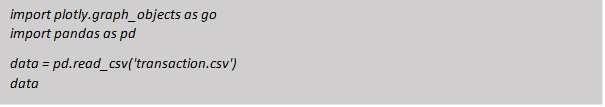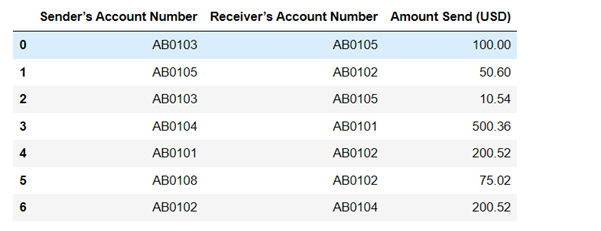After reading the data as pandas data frame we need to give it a structure which can be used to make the graph.

Plotly library will be used to create the graph and for that, we need to have four lists-
1. Label
2. Source
3. Target
4. Value.

Now Label contains all the unique node names.
Source contains positional index of the source nodes from the label.
Target contains positional index of the target nodes from the label
and Value contains all the values corresponding to each source and target node index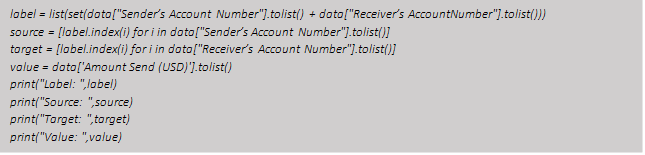Let’s create all those lists.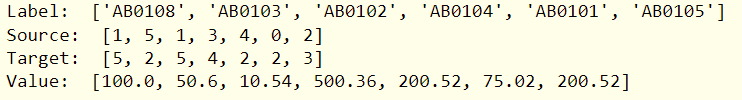As we can see, Label is having all the unique account id. Source and Target are having all the indexes. For example, in the 1st row we are having Source -> AB0103 and Target -> AB0105 and Value -> 100.0. Therefore, index position for AB0103 is 1 and for AB0105 is 5 in Label list, hence the 1st element of Source and Target is 1 and 5 respectively and for Value is 100.0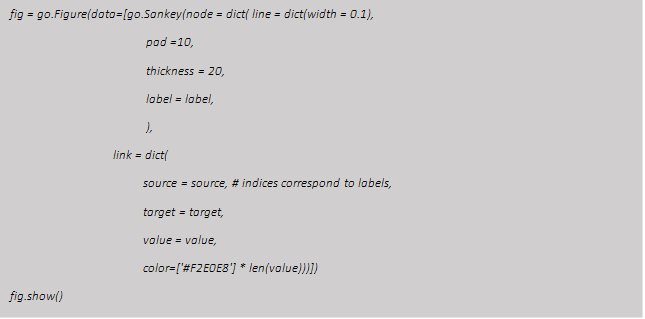Now all set, we just need to use these 4 lists to build the plot as below.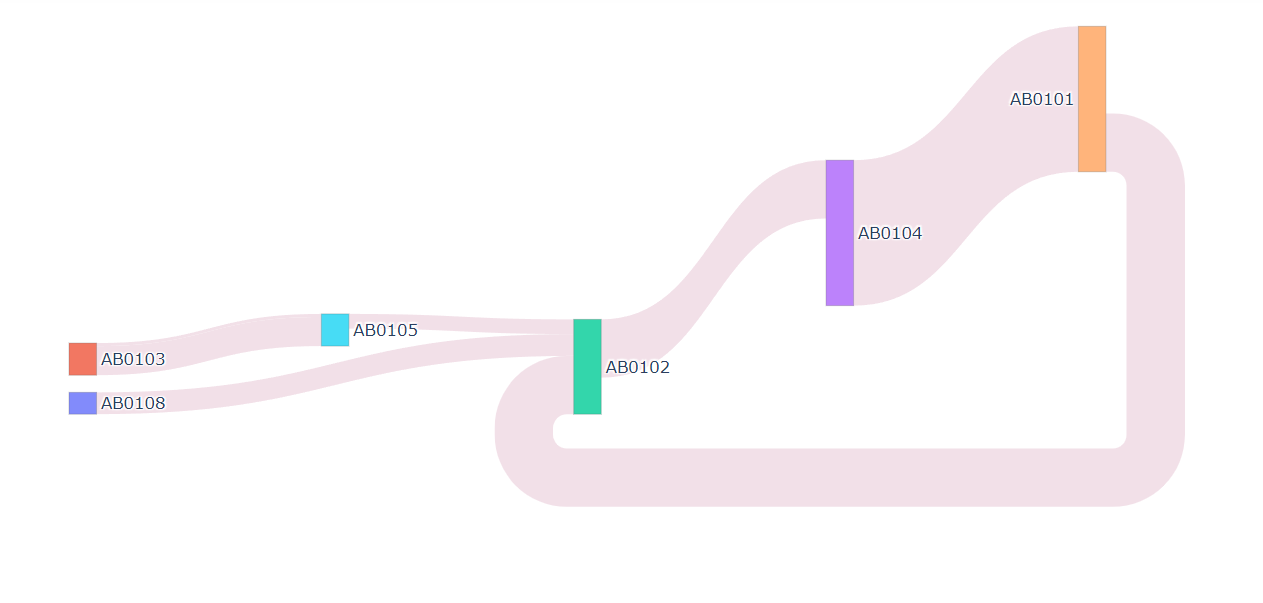While hovering across the nodes or flows, we can see the properties of nodes or flows. Refer to the below images for more information.

### Other use cases

Here I am showing one of the use cases, where we can use Sankey plot. There are other use cases where we can use Sankey plot, like

1. Black Money tracking: Sankey can help in tracking money through accounts in either direction, thus help to track black money.

2. Social Media Connection Tracking: Sankey also can be used in tracking social media people’s connections.

3. Bug Tracking: For a particular bug, we can track its starting and ending point using Sankey.

### Conclusion

Sankey can be used in various use cases. Mostly, any kind of Graph Network can be visually shown by Sankey plot. Moreover, using python we can easily make a Sankey plot and also, we can implement that with our daily business needs.

References